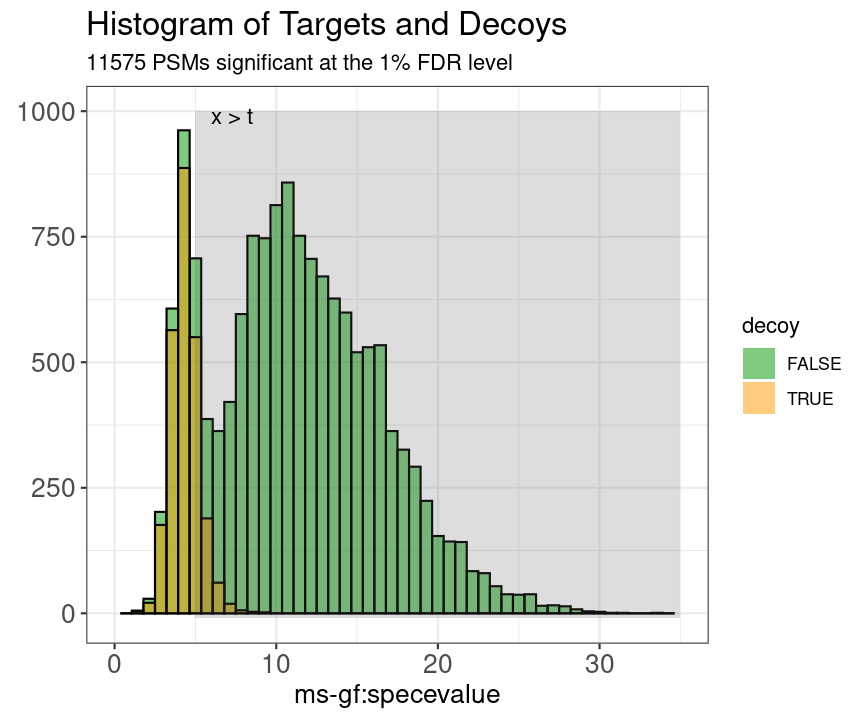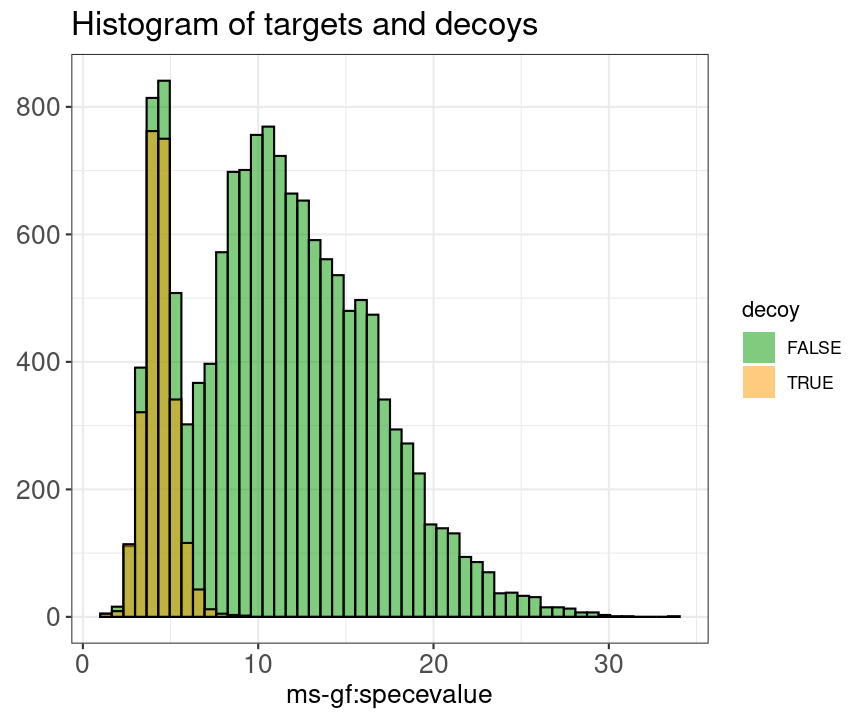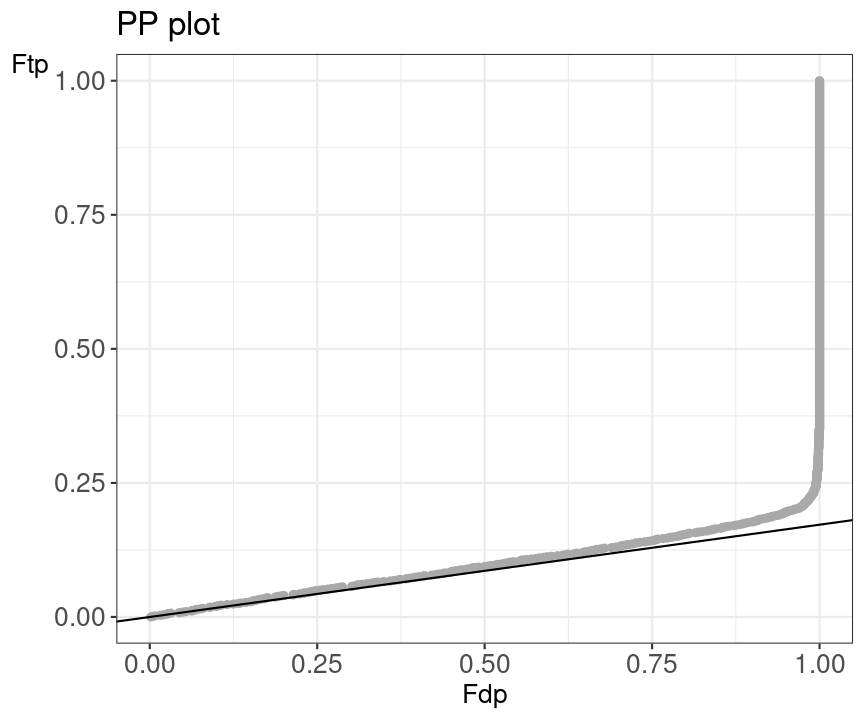library(TargetDecoy)
library(ggplot2)

# 1 Basics

## 1.1 Installing TargetDecoy

TargetDecoy is an R package available via the Bioconductor repository for packages. R can be installed on any operating system from CRAN after which you can install TargetDecoy by using the following commands in your R session:

if (!requireNamespace("BiocManager", quietly = TRUE)) {
install.packages("BiocManager")
}

BiocManager::install("TargetDecoy")

## Check that you have a valid Bioconductor installation
BiocManager::valid()

The latest development version of TargetDecoy can be installed from GitHub by running the following in an R session:

BiocManager::install("statOmics/TargetDecoy")

## 1.2 Citing TargetDecoy

We hope that TargetDecoy will be useful for your research. Please use the following information to cite the package and the overall approach. Thank you!

## Citation info
citation("TargetDecoy")
#>
#> To cite package 'TargetDecoy' in publications use:
#>
#>   Debrie E, Clement L, Malfait M (2022). _TargetDecoy: Diagnostic Plots
#>   to Evaluate the Target Decoy Approach_.
#>   https://www.bioconductor.org/packages/TargetDecoy,
#>   https://statomics.github.io/TargetDecoy/,
#>   https://github.com/statOmics/TargetDecoy/.
#>
#> A BibTeX entry for LaTeX users is
#>
#>   @Manual{,
#>     title = {TargetDecoy: Diagnostic Plots to Evaluate the Target Decoy Approach},
#>     author = {Elke Debrie and Lieven Clement and Milan Malfait},
#>     year = {2022},
#>     note = {https://www.bioconductor.org/packages/TargetDecoy, https://statomics.github.io/TargetDecoy/, https://github.com/statOmics/TargetDecoy/},
#>   }

# 2 Introduction

A first step in the data analysis of Mass Spectrometry (MS) based proteomics data is to identify peptides and proteins. For this, a huge number of experimental mass spectra typically have to be assigned to theoretical peptides derived from a sequence database. This task is carried out by specialised algorithms called search engines, which compare each of the observed spectra to theoretical spectra derived from relevant candidate peptides obtained from a sequence data base and calculate a score for each comparison. The observed spectrum is then assigned to the theoretical peptide with the best score, which is also referred to as a peptide-to-spectrum match (PSM). It is of course crucial for the downstream analysis to evaluate the overall reliability of these matches. Therefore False Discovery Rate (FDR) control is used to return a sufficiently reliable list of PSMs. The FDR calculation, however, requires a good characterisation of the score distribution of PSMs that are matched to an incorrect peptide (bad target hits). In proteomics, the target decoy approach (TDA) is typically used for this purpose. The TDA method matches the observed spectra to a database of real (target) and nonsense (decoy) peptides, with the latter typically generated by reversing protein sequences in the target database. Hence, all PSMs that match to a decoy peptide are known to be bad hits and the distribution of their scores is used to estimate the distribution of the bad scoring target PSMs. A crucial assumption of the TDA is that decoy PSM hits have similar properties as bad target hits so that decoy PSM scores are a good simulation of target PSM scores. Users, however, typically do not evaluate these assumptions. To this end we developed TargetDecoy to generate diagnostic plots to evaluate the quality of the target decoy method, thus allowing users to assess whether the key assumptions underlying the method are met.

# 3 Concepts

## 3.1 Basic Statistical Concepts

We first introduce some notation. With $$x$$ we denote the PSM and without loss of generality we assume that larger score values indicate a better match to the theoretical spectrum. The scores will follow a mixture distribution:

$f(x) = \pi_0f_0(x)+(1-\pi_0)f_1(x),$

with $$f(x)$$ the target PSM score distribution, $$f_0(x)$$ the mixture component corresponding to incorrect PSMs, $$f_1(x)$$ the mixture component corresponding to the correct PSMs and $$\pi_0$$ the fraction of incorrect PSMs. Based on the mixture distribution we can calculate the posterior probability that a PSM with score $$x$$ is a bad match:

$P[\text{Bad hit} \mid \text{score }x]=\frac{\pi_0 f_0 (x)}{f(x)},$

which is also referred to as the posterior error probability (PEP) in mass spectrometry based proteomics. With the mixture model, we can also calculate the posterior probability that a random PSM in the set of all PSMs with scores above a score threshold t is a bad hit:

$P[\text{Bad hit} \mid \text{score }x>t]=\pi_0 \frac{\int\limits_{x=t}^{+\infty} f_0(x)dx}{\int\limits_{x=t}^{+\infty} f(x)dx},$

with $$\int\limits_{x=t}^{+\infty} f_0(x) dx$$ the probability to observe a bad PSM hit above the threshold and, $$\int\limits_{x=t}^{+\infty} f(x) dx$$ the probability to observe a target PSM hit above the threshold. The probability $$P[\text{Bad hit} \mid \text{score }x>t]$$ is also referred to as the False Discovery Rate for peptide identification (FDR) of the set of PSMs with scores above the threshold $$t$$. Hence, the FDR has the interpretation of the expected fraction of bad hits in the set of all target hits that are returned in the final PSM list, so bad PSM hits with scores above the threshold.

In order to estimate the FDR, we thus have to estimate the distribution of the bad hits and of the targets. In proteomics this is typically done by the use of the Target Decoy Approach (TDA).

## 3.2 Target Decoy Approach

A competitive target decoy search involves performing a search on a concatenated target and decoy database (as, for example, obtained from a reversed target database). Typically, one will ensure that there are as many targets as there are decoys. If the decoy hits are a good simulation of the bad target hits, it is equally likely that a bad hit will go to the targets as as it is to go to the decoys. With a competitive target decoy approach, it is therefore assumed that a bad hit matches a bad target equally likely as a decoy.

The distribution of bad target hits ($$f_0(t)$$) and the marginal distribution of all target hits ($$f(t)$$) is empirically estimated using the decoy scores and all target scores respectively. With the TDA, the FDR of the set of returned PSMs with scores above a threshold t, is estimated by dividing the number of decoy hits with a score above t by the number of target PSMs with a score above t.

$\widehat{\text{FDR}}(t) = \frac{\#\widehat{\text{ bad hits}} \mid x>t}{\#\text{ targets} \mid x>t} \stackrel{(*)}{=} \frac{\#\text{ decoys} \mid x>t}{\#\text{ targets} \mid x>t} \\ (*) \text{ Assumption TDA}: \text{bad targets} \stackrel{d}{=} \text{decoys}$

This can be rewritten as:

$\widehat{\text{FDR}}(t)=\frac{\#decoys}{\#targets} \cdot \frac{\frac{\# decoys \mid x>t}{\#decoys}}{\frac{\#targets \mid x>t}{\#targets}}$

$\widehat{\text{FDR}}(t) = {\widehat{\pi}}_0 \frac{\widehat{\int\limits_t^{+\infty} f_0(x) dx}}{\widehat{\int\limits_t^{+\infty} f(x)dx}}$

Hence, the proportion of bad hits $$\pi_0$$ is estimated as the number of decoys divided by the number of targets, since the competitive TDA assumes that it is equally likely that a bad hit matches to a bad target hit or to a decoy. The probability on a (bad) target PSM hit above the threshold is estimated based on the empirical cumulative distribution in the sample, i.e. as the fraction of targets (decoys) that are above the threshold. Hence, a second assumption is that the decoy scores provide a good simulation of the bad target scores.Figure 1: Illustration of the target and decoy distributions
The green bars are the histogram of the target PSM scores. The orange bars are the histogram of the decoy PSM scores. We see that the decoy PSMs match well with the incorrect target hits that are more likely to occur at low scores.

In summary, two assumptions have to be checked:

• Assumption 1: The decoy PSM hits are a good simulation of the bad target hits and the distributions are equal.

• Assumption 2: When the library size of targets and decoys is the same, it is equally likely that a bad hit matches to a target sequence or to a decoy sequence.

When these two assumptions are met we can replace the number of bad hits by the number of decoys.

These assumptions can be checked using a histogram and a PP-plot.

# 4 Diagnostic plots

## 4.1 Histogram

In the histogram the shape of the score distribution of the decoys (shown in blue in the figure below) should be equal to that of bad target hits (first mode in the target distribution indicated in red). Note that the height of the decoys can be slightly lower than the first mode in the target distribution because some good target hits also have a low score (e.g., because of poor quality observed spectra).

data("ModSwiss")
evalTargetDecoysHist(ModSwiss,
decoy = "isdecoy", score = "ms-gf:specevalue",
log10 = TRUE, nBins = 50
)Figure 2: Histogram of the scores of targets and decoys

## 4.2 PPplot

The figure below shows an example of a PP plot, the main diagnostic plot to evaluate the quality of the decoys.

evalTargetDecoysPPPlot(ModSwiss,
decoy = "isdecoy", score = "ms-gf:specevalue",
log10 = TRUE
)Figure 3: PP plot

Deviations from the assumptions of TDA can be best evaluated in the PP-plot. The PP-plot displays the empirical cumulative distribution (ECDF) from the target distribution in function of that of the decoy distribution. PP-plots have the property that they show a straight 45 degree line through the origin if and only if both distributions are equivalent. Any deviation from this straight line indicates that the distributions differ. Note that we expect that these distributions will differ for targets and decoys. Indeed, the target distribution is stochastically larger than the decoy distribution as larger scores indicate more reliable hits and as decoys are believed to behave similarly to bad target hits. Hence, the PP-plot for targets versus decoys will always be expected to lie below the 45 degree line. When the decoys are a good simulation for the bad target hits, however, the lower values in the PP-plot should lie on a straight line through the origin with a slope determined by $$\widehat{\pi}_0$$. Indeed, small target PSM scores are most likely bad hits. Hence we will include the $$\widehat{\pi}_0$$-line in our diagnostic PP plot to evaluate the quality of the decoys.

When the assumptions of the TDA approach are violated, the dots in the PP-plot at lower percentiles will deviate from the $$\widehat{\pi}_0$$ line. In case the PP-plot is still a straight line at lower percentiles, then the shape of the decoy distribution is correct, but there are less (or more) decoys than expected under the concatenated TDA assumption, which could occur if the decoy database is different in size than the target database or when a bad hit is less likely to match to a decoy than to a bad target hit. This would also be visible in the histograms: the decoy histogram would be considerably lower (higher) than the first mode of the target distribution. When the PP-plot at lower percentiles deviates from a straight line, the distribution of decoys and the bad target PSMs is not equivalent, indicating that the decoys are not a good simulation of bad target hits. Either type of deviation should be of concern as each indicates that the FDR returned by the conventional concatenated TDA is incorrect.

# 5 Supported data formats

TargetDecoy currently supports objects of class mzID or mzRIdent from the mzID and mzR packages, respectively.

These datasets are typically loaded from an .mzid file as follows

filename <- system.file("extdata/55merge_omssa.mzid", package = "mzID")

## mzID
mzID_object <- mzID::mzID(filename)
class(mzID_object)
#>  "mzID"
#> attr(,"package")
#>  "mzID"

## mzRIdent
mzR_object <- mzR::openIDfile(filename)
class(mzR_object)
#>  "mzRident"
#> attr(,"package")
#>  "mzR"

# 6 Examples

## 6.1 The data

In this vignette we use data from a Pyrococcus furiosis sample run on a LTQ-Orbitrap Velos mass spectrometer. The data can be found in the PRIDE repository with identifier PXD001077. The Pyrococcus furiosis reference proteome fasta files were downloaded from UniProtKB and UniProtKB/Swiss-Prot on April 22, 2016. The Pyrococcus data was searched against all Pyrococcus proteins with multiple search engines using either the reference proteome from UniProtKB or the reference proteome from UniProtKB/Swiss-Prot.

This dataset is available in the TargetDecoy installation and can be loaded with data("ModSwiss").

## 6.2 Example 1: One file and one search engine

### 6.2.1 Example 1.1: MSGF+ search engine

In the next example MS-GF+ was used as the database search engine and SwissProt was used as protein sequence database. The MS-GF+ search provided an mzid file.

## Load the example SwissProt dataset
data("ModSwiss")

We use the function evalTargetDecoys(). This function creates two diagnostic plots, i.e. a histogram and a PP plot. It can be used to evaluate the quality of the target decoy approach, i.e. to check the competitive TDA assumptions.

It has the following arguments:

• object: mzID object, mzR object or data.frame
• decoy: Character, name of the decoy variable which consists of a boolean that indicates if the score belongs to a target or a decoy. Name of this variable in the file (typically "isdecoy" or "isDecoy").
• score: Score variable contains the scores of the search engine, which have to be continuous (larger scores are assumed to be better. E-values are typically -log10(e-value) transformed.) Name of the variable depends on the search engine.
• log10: Logical. Should the score be log10 transformed? Typically used when the score is an e-value. The default is TRUE.
• nBins: Number of bins in the histogram. The default is 50

evalTargetDecoys() will return a figure consisting of 4 plots:

1. A PP plot showing the empirical cumulative distribution of the target distribution in function of that of the decoy distribution
2. A histogram showing the score distributions of the decoys and non-decoys
3. A zoomed PP plot
4. A zoomed histogram
evalTargetDecoys(ModSwiss,
decoy = "isdecoy", score = "ms-gf:specevalue",
log10 = TRUE, nBins = 50
)